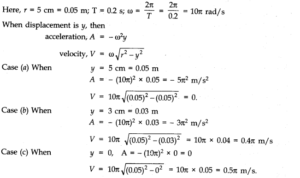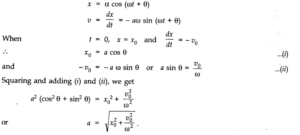# Chapter 14 – Oscillations Questions and Answers: NCERT Solutions for Class 11 Physics

Class 11 Physics NCERT book solutions for Chapter 14 - Oscillations Questions and Answers.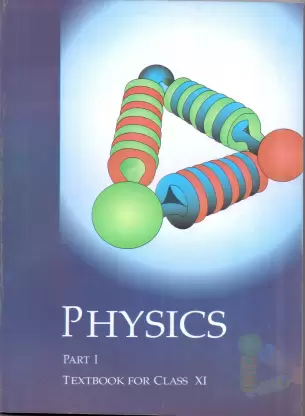## Question 14. 3. Fig. depicts four x-t plots for linear motion of a particle. Which of ike plots represent periodic motion? What is the period of motion (in case of periodic motion)?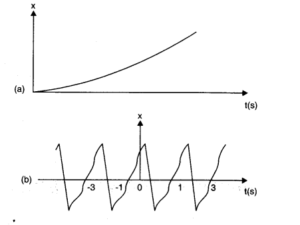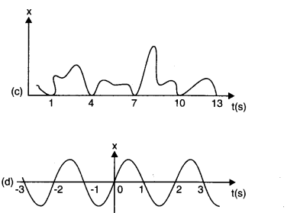## Question 14. 4. Which of the following function of time represent (a) simple harmonic, (b) periodic but not simple harmonic, and (c) non-periodic motion? Give period for each case of periodic motion (to is any positive constant). (a) sin wt – cos wt (b) sin2wt (c) 3 cos -2 cos (π/4-2 wt) (d) cos wt + cos 3 wt + cos 5 wt (e) exp (- w2t2) (f) 1 + wt + w2t2.

### Answer:The function will represent a periodic motion, if it is identically repeated after a fixed interval of time and will represent S.H.M if it can be written uniquely in the form of a cos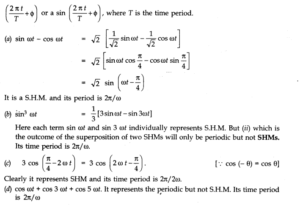## Question 14. 5. A particle is in linear simple harmonic motion between two points, A and B, 10 cm apart. Take the direction from A to B as the positive direction and give the signs of velocity, acceleration and force on the particle when it is (a) at the end A, (b) at the end B, (c) at the mid-point of AB going towards A, (d) at 2 cm away from B going towards A, (e) at 3 cm away from A going towards B, and (f) at 4 cm away from B going towards A.

### Answer:In the fig. (given below), the points A and B, 10 cm apart, are the extreme positions of the particle in SHM, and the point O is the mean position. The direction from A to B is positive, as indicated.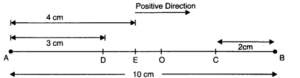## Question 14. 7. The motion of a particle executing simple harmonic motion is described by the displacement function.x(t) = A cos (wt +Ф ).If the initial (t = 0) position of the particle is 1 cm and its initial velocity is w cm/s, what are its amplitude and initial phase angle? The angular frequency of the particle is π s-1. If instead of the cosine function, we choose the sine function to describe the SHM: x = B sin (wt + α), what are the amplitude and initial phase of the particle with the above initial conditions?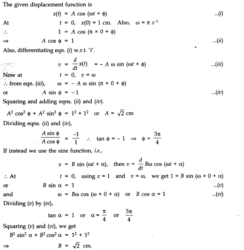## Question 14. 8. A spring balance has a scale that reads from 0 to 50 kg. The length of the scale is 20 cm. A body suspended from this balance, when displaced and released, oscillates with a period of 0.6 s. What is the weight of the body?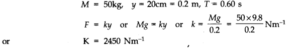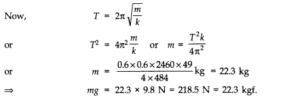## Question 14. 9. A spring having with a spring constant 1200 Nm-1is mounted on a horizontal table as shown in Fig. A mass of 3 kg is attached to the free end of the spring. The mass is then pulled sideways to a distance of 2.0 cm and released. Determine (i) the frequency of oscillations, (ii) maximum acceleration of the mass, and (iii) the maximum speed of the mass.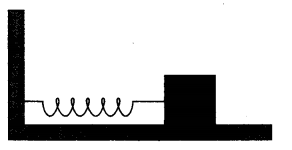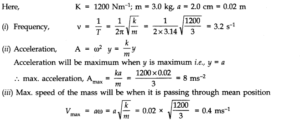## Question 14. 10. In Exercise 9, let us take the position of mass when the spring is unstreched as x = 0, and the direction from left to right as the positive direction of x – axis. Give x as a function of time t for the oscillating mass if at the moment we start the stopwatch (t = 0), the mass is(a)at the mean position,(b)at the maximum stretched position, and(c)at the maximum compressed position.In what way do these functions for SHM differ from each other, in frequency, in amplitude or the initial phase?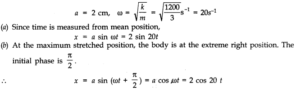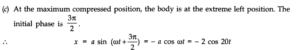## Question 14. 11. The following figures correspond to two circular motions. The radius of the circle, the period of revolution, the initial position, and the sense of revolution (i.e., clockwise or anticlockwise) are indicated on each figure.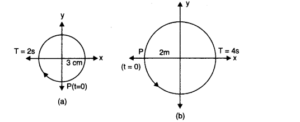## Obtain the corresponding simple harmonic motions of the x-projection of the radius vector of the revolving particle P in each case.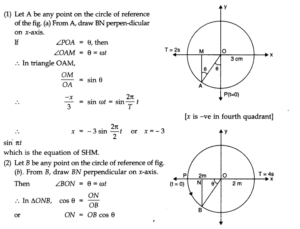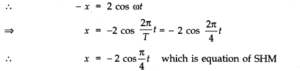## Question 14. 12. Plot the corresponding reference circle for each of the following simple harmonic motions. Indicate the initial (t = 0) position of the particle, the radius of the circle, and the angular speed of the rotating particle. For simplicity, the sense of rotation may be fixed to be anti-clockwise in every case: (x is in cm and t is in s) (a) x = – 2 sin (3t + π /3) (b) x = cos (π /6 – t) (c) x = 3 sin (2πt + π /4) (d) x = 2 cos π t.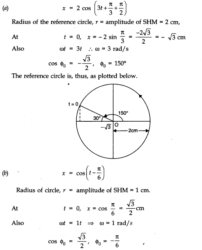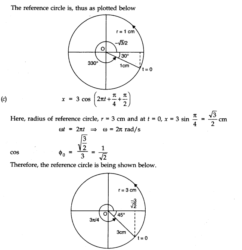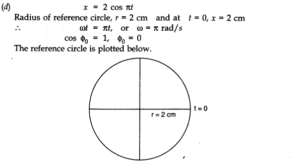## Question 14. 13. Figure (a) shows a spring of force constant k clamped rigidly at one end and a mass m attached to its free end. A force F applied at the free end stretches the spring. Figure (b) shows the same spring with both ends free and attached to a mass m at either end. Each end of the spring in Figure – (b) is stretched by the same force F.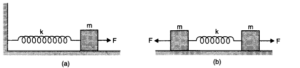## (a) What is the maximum extension of the spring in the two cases? (b) If the mass in Fig. (a) and the two masses in Fig. (b) are released free, what is the period of oscillation in each case?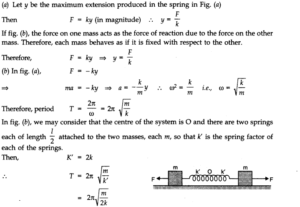## Question 14. 14. The piston in the cylinder head of a locomotive has a stroke (twice the amplitude) of 1.0 m. If the piston moves with simple harmonic motion with an angular frequency of 200 rev/min, what its maximum speed?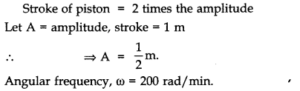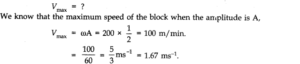## Question 14. 15. The acceleration due to gravity on the surface of moon is 1.7 ms-2. What is the time period of a simple pendulum on the surface of moon if its time-period on the surface of Earth is 3.5 s? (g on the surface of Earth is 9.8 ms-2.)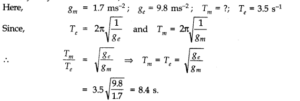## Question 14. 16.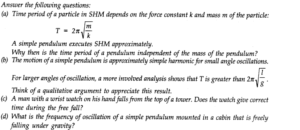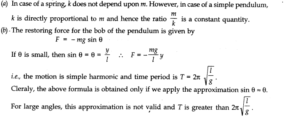## Question 14. 17. A simple pendulum of length l and having a bob of mass M is suspended in a car. The car is moving on a circular track of radius R with a uniform speed v. If the pendulum makes small oscillations in a radial direction about its equilibrium position, what will be its time period?

### Answer:In this case, the bob of the pendulum is under the action of two accelerations.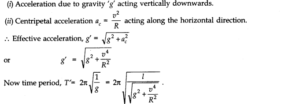## Question 14. 18. A cylindrical piece of cork of density of base area A and height h floats in a liquid of density ρ1. The cork is depressed slightly and then released. Show that the cork oscillates up and down simple harmonically with a period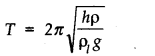## where ρ is the density of cork. (Ignore damping due to viscosity of the liquid).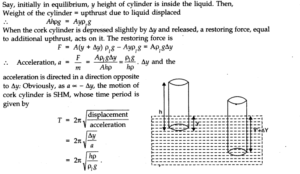## Question 14. 19. One end of a U-tube containing mercury is connected to a suction pump and the other end to the atmosphere.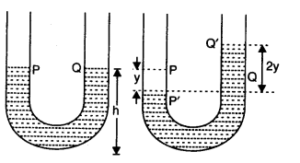## A small pressure difference is maintained between the two columns. Show that, when the suction pump is removed, the column of mercury in the U-tube executes simple harmonic motion.

### Answer:The suction pump creates the pressure difference, thus mercury rises in one limb of the U-tube. When it is removed, a net force acts on the liquid column due to the difference in levels of mercury in the two limbs and hence the liquid column executes S.H.M. which can be expressed as: Consider the mercury contained in a vertical U-tube upto the level P and Q in its two limbs. Let P = density of the mercury. L = Total length of the mercury column in both the limbs. A = internal cross-sectional area of U-tube. m = mass of mercury in U-tube = LAP. Assume, the mercury be depressed in left limb to F by a small distance y, then it rises by the same amount in the right limb to position Q’. .’. Difference in levels in the two limbs = P’ Q’ = 2y. :. Volume of mercury contained in the column of length 2y = A X 2y .•. m – A x 2y x ρ. If W = weight of liquid contained in the column of length 2y. Then W = mg = A x 2y x ρ x g This weight produces the restoring force (F) which tends to bring back the mercury to its equilibrium position.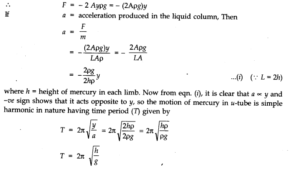## Question 14. 20. An air chamber of volume V has a neck area of cross-section into which a ball of mass m just fits and can move up and down without any friction (Fig.). Shaw that when the ball is pressed down a little and released, it executes SHM. Obtain an expression for the time period of oscillations assuming pressure-volume variations of air to be isothermal.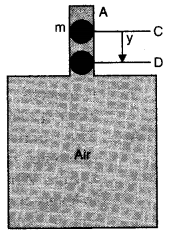### Answer:Consider an air chamber of volume V with a long neck of uniform area of cross-section A, and a frictionless ball of mass m fitted smoothly in the neck at position C, Fig. The pressure of air below the ball inside the chamber is equal to the atmospheric pressure. Increase the pressure on the ball by a little amount p, so that the ball is depressed to position D, where CD = y. There will be decrease in volume and hence increase in pressure of air inside the chamber. The decrease in volume of the air inside the chamber, ΔV = Ay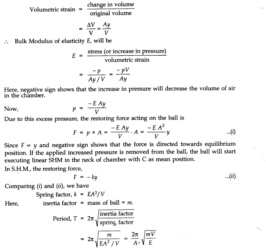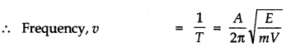## Question 14. 21. You are riding an automobile of mass 3000 kg. Assuming that you are examining the oscillation characteristics of its suspension system. The suspension sags 15 cm when the entire automobile is placed on it. Also, the amplitude of oscillation decreases by 50% during one complete oscillation. Estimate the values of (a) the spring constant k and (b) the damping constant b for the spring and shock absorber system of one wheel, assuming that each wheel supports 750 kg. g = 10 m/s2.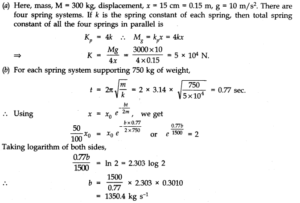## Question 14. 22. Show that for a particle in linear SHM the average kinetic energy over a period of oscillation equals the average potential energy over the same period.

### Answer:Let the particle executing SHM starts oscillating from its mean position. Then displacement equation is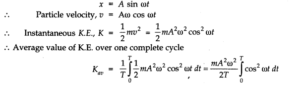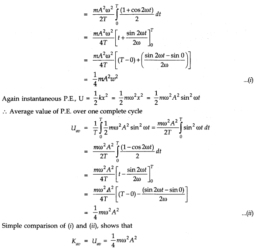## Question 14. 23. A circular disc, of mass 10 kg, is suspended by a wire attached to its centre. The wire is twisted by rotating the disc and released. The period of torsional oscillations of found to be 1.5 s. The radius of the disc is 15 cm. Determine the torsional spring constant of the wire. (Torsional spring constant) a is defined by the relation J = – αθ, where J is the restoring couple and θ the angle of twist).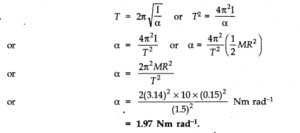## Question 14. 24. A body describes simple harmonic motion with an amplitude of 5 cm and a period of 0.2 s. Find the acceleration and velocity of the body when the displacement is (a) 5 cm (b) 3 cm (c) 0 cm